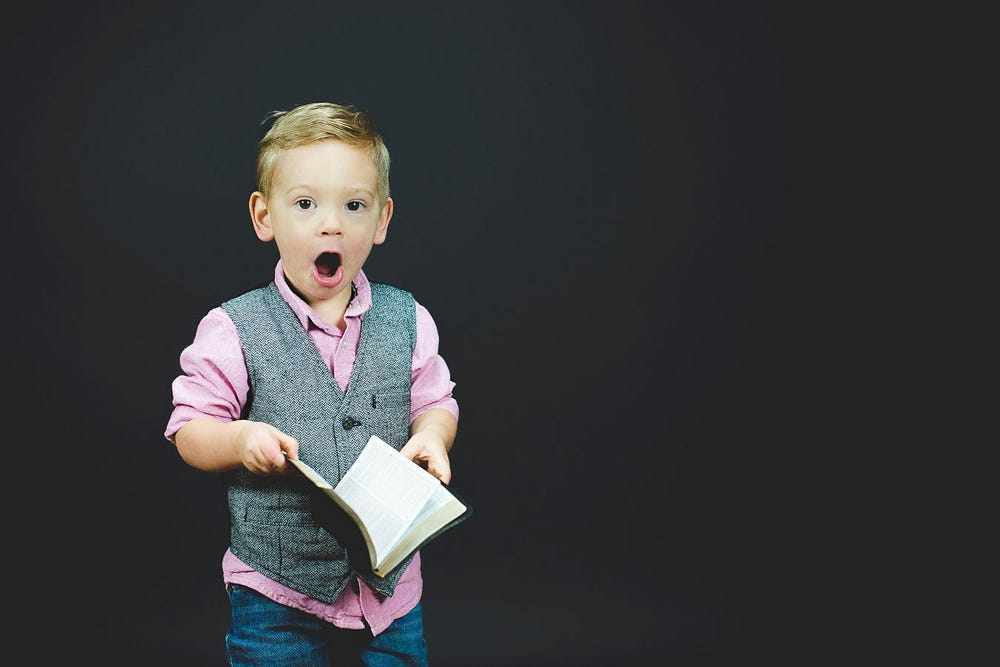# Data Science Course 6/10: Classification & Supervised Learning

Source: Deep Learning on Medium# Data Science Course 6/10: Classification & Supervised Learning

## Let’s learn Data Science in 2020

This is the 6th instalment of Data Science Crash Course. We have already learned about storing data and where to get data from. In this lecture I will cover standard techniques for classifying data, which is the basic application of Data Science.

# What is Supervised Learning

Imagine you have data with labels attached. Think images of animals with a description whether it’s a cat or a dog (classification problem). Another example is data about customers in an ecommerce with information like age group, occupation, past shopping (regression problem). Supervised learning deals with this type of problems, where you have labels attached to data so that you can ‘supervise’ the learning process of your algorithms using those labels as a guidance.

Classification is the problem of identifying to which label (category) a new object belongs, on the basis of a training set of data containing objects whose labels are known. Examples are assigning a given email to the “spam” or “non-spam” class, and assigning a diagnosis to a given patient based on observed characteristics of the patient.

Regression is a statistical process of estimating a value (‘continuous label’) based on other features or variables.

# Classification and Regression Algorithms

K-Nearest Neighbours (KNN) is the most standard example for both classification and regression. We’re looking at objects closest to a given example we start with and attach a label based on that. K stands for a number of neighbours we’re looking at. Have a look at this implementation of KNN, when K=2, using sklearn, where we want to understand 6 points on a plane:

Naive Bayes is a standard approach. You simplify a situation and assume that actions/objects in your data are independent (in a probabilistic sense) and hence you can compute probabilities using Bayes theorem. Formally “Naive Bayes methods are a set of supervised learning algorithms based on applying Bayes’ theorem with the “naive” assumption of conditional independence between every pair of features given the value of the class variable.” You can implement it using sklearn again, for example with this example using a standard dataset of irises and modeling conditional probability using normal distribution (GaussianNB):

Regression is used when you try to predict a precise value of a label. So it’s a continuous variant of a classification problem. This would be perfect for trying to determine an income of a person based on people living close to him.

Linear regressions is the simplest — where you assume your data can be modeled by a linear function. There are plethora of regressions modeled after various functions, the most popular being sigmoid and ReLu (rectified linear function).

Again Linear Regression is easy to implement with sklearn. Have a look at this tutorial to learn about using Linear Regression with Python.

Another classification technique is decision trees, where you try to have a Q&A format of data like:

• does this person live in a flat or a house?
• is this person over thirty?
• does this person have kids?

Trees can be built automatically. For example you can build a simple decision tree to divide the plane into two regions using sklearn:

Building trees is great because then you can join a couple of trees into a larger model via voting. Decision of each decision tree is taken into account and all decisions are weighted (arithmetic mean in the simplest case). This kind of methods are called ensemble learning.